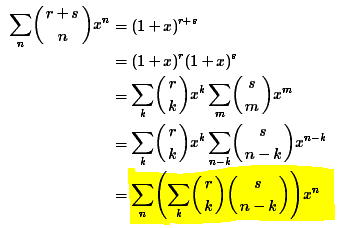# Problem Related to Binomial Coefficient

dpa
Hi All,

## Homework Statement

This is algebraic proof of Vandermonde's identity:

I am having some problem understanding how we reached the second last step and more importantly, last steps from revious steps.src::proofwiki.org

I would be grateful if someone would elaborate it clearly.

## Homework Equations

Basically none that are relevant to answer my question.

## The Attempt at a Solution

I have no idea how we reached the last two steps.

Thank You.

Last edited:

Homework Helper
Gold Member
Hi All,

## Homework Statement

This is algebraic proof of Vandermonde's identity:

I am having some problem understanding how we reached the second last step and more importantly, last steps from revious steps.src::proofwiki.org

I would be grateful if someone would elaborate it clearly.

## Homework Equations

Basically none that are relevant to answer my question.

## The Attempt at a Solution

I have no idea how we reached the last two steps.

Thank You.

It is no wonder you are having trouble following that. Besides having a typo, it is written very sloppily. You are working on this (in which I have included the limits):$$\sum_{k=0}^r \binom r k x^k\sum_{m=0}^s \binom s m x^m$$Notice it started with summation over a rectangle in the ##m,k## plane: ##0\le k\le r,\,0\le m\le s##.

In the second sum he makes a change of index from ##m## to ##n## by substituting ##n=m+k## or ##m=n-k##. This gives, including the limits:$$\sum_{k=0}^r\binom r k x^k\sum_{n=k}^{s+k}\binom s {n-k}x^{n-k}$$Notice that the lower limit on the second sum is ##n=k##, not ##n-k##. That is a typo. Notice that the region of summation is now ##k\le n\le s+k,\, 0\le k \le r##. If you draw that region in the ##n,k## plane you will see it is a parallelogram. And he has ignored the upper limits in the sums.

What he does next is put it all under a double sum and reverse the order of summation. But when you reverse the limits it gets complicated putting the limits on in the other order. You would have to break the region up into two sums. That is probably why he has ignored the upper limits in the writeup. Good luck deciphering the rest of that writeup.

dpa
Thank You. That was very very helpful.

dpa
My goal actually is simpler. I have to prove
($\stackrel{2n}{k}$)=$\Sigma^{k}_{l=0}$($\stackrel{n}{l}$)($\stackrel{n}{k-l}$)

I can't properly use latex but hope you can understand it.

I wanted to follow the above pattern to prove it, but if there is a simpler method, that would be helpful.

Thank You.

Homework Helper
My goal actually is simpler. I have to prove
($\stackrel{2n}{k}$)=$\Sigma^{k}_{l=0}$($\stackrel{n}{l}$)($\stackrel{n}{k-l}$)

I can't properly use latex but hope you can understand it.

I wanted to follow the above pattern to prove it, but if there is a simpler method, that would be helpful.

Thank You.

It's pretty easy to give a combinatorial argument. You want to chose k objects from 2n objects. Split the 2n up into two groups n. Count the number of ways to pick l from the first group and l-k from the other and sum them up.

dpa
Hi Dick,

Thank you for your suggestion. I am sorry for not including some point. I am actually looking for algebraic proof.

Thank You.